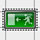bontegamesHi! I'm Bart Bonte, a Belgian independent game designer and bontegames.com is where I blog about new interesting browser and mobile games. My own games are all in the left column (or at the bottom of this page on mobile). More info about me and my games on bartbonte.com.

## November 13, 2007

### excitExcit definitely qualifies as the best safe for workspace puzzle game ever. You're trapped in a spreadsheet and you need to escape the different levels using the cursor keys without sliding of the screen. Excit was developed by Creative Units.

Unknown said...

Well, that's a nice game, but it is a copy from some other game, which name I don't remember =(( The original surely had a better design.
And this one is easier.Anonymous said...

i cant pass level 23 =[Anonymous said...

Here's a full excit solution or 'walkthrough', have fun!

level 1: (Numbers) D L D
level 2: (Solution) R U L U
level 3: (Business) D R U
level 4: (Management) R U R L U R
level 5: (Detail) L U R D L R D U R L
level 6: (Report) U D R D U L D R D L D U
level 7: (Plain) D L R U L D R L R D R U
level 8: (Opportunities) U R U L D U R U L U R L
level 9: (Workflow) R L D R U R D L U L U R D L D R U L D U R U
level 10: (Simulation) R L D U R L D R U L R Ulevel 11: (Strategy) R L D L U R D R U L U
level 12: (Environment) L D R U R U R
level 13: (Knowledge) R U L D R D R U L D L U L U D R D L U L D R U L D L R
level 14: (Productivity) L D R U R L D L U R D L D L U R D R U
level 15: (Analysis) R U R D R U D L U R L D R D U R L
level 16: (Integration) L D L D L R D L R D U D R L D
level 17: (Reporting) L U R D R U L R D R U R D R U D L D R U L D R L R D L U R D L D L U
level 18: (Forecasting) D L U L R D R U R D L R L D R D R U L
level 19: (Budgeting) L D L R L U L R U R D L D L D R D U L D R R D R D U
level 20: (Consolidation) U R D L D R U R U L D R D R U R D R U R D L D R D R U R D R U L R U L
level 21: (Scalability) L D R D R U L D R U L D R U L D R U
level 22: (Framework) D L U R D R L U D R U L D L
level 23: (Planning) R R D U R U R D L U R D L D U R U R D L U R D R U L D R U L U L D R
level 24: (MIS Alea) U L D L U R U D L D R U L U R U R D L D R D R D L D L U L R U L D L R D L D L U R L D
level 25: (MIS onVision) R R U R D R D R D U D L L D R U R U L U R L D U L U L U D L U R U D R
level 26: (MIS DeltaMiner) L U D R D L D L D R U L D U L R D U L U R L U R D L D L
level 27: (Subsidary) R U D R U R U R D L U R L
level 28: (CIO) D R D L D R D U U L R U U R U L D R D R D L L U R D U R D L D L U L R D L D R D L D R D R U
level 29: (CFO) R D L D L U R U R D L U L U D L U R D L R U R D R R L R D L D L U R U L D L D R U L U L D L U R D R U R D R R D L D L U R U R D
level 30: (Goal) D U D R L D R L D L R D U D L R U L D U L D L D L R D R U L U R D U L U R D L U L U R D L U R D R U D L D L R L R U L UAnonymous said...

That game you're thinking of was probably Orbox, very simalar conceptAnonymous said...

level 26 dosent work for me nozemNat R. said...